Test: Motion- Case Based Type Questions- 2

# Test: Motion- Case Based Type Questions- 2 - Class 9

Test Description

## 10 Questions MCQ Test Physics for Class 9 - Test: Motion- Case Based Type Questions- 2

Test: Motion- Case Based Type Questions- 2 for Class 9 2023 is part of Physics for Class 9 preparation. The Test: Motion- Case Based Type Questions- 2 questions and answers have been prepared according to the Class 9 exam syllabus.The Test: Motion- Case Based Type Questions- 2 MCQs are made for Class 9 2023 Exam. Find important definitions, questions, notes, meanings, examples, exercises, MCQs and online tests for Test: Motion- Case Based Type Questions- 2 below.
Solutions of Test: Motion- Case Based Type Questions- 2 questions in English are available as part of our Physics for Class 9 for Class 9 & Test: Motion- Case Based Type Questions- 2 solutions in Hindi for Physics for Class 9 course. Download more important topics, notes, lectures and mock test series for Class 9 Exam by signing up for free. Attempt Test: Motion- Case Based Type Questions- 2 | 10 questions in 20 minutes | Mock test for Class 9 preparation | Free important questions MCQ to study Physics for Class 9 for Class 9 Exam | Download free PDF with solutions
 1 Crore+ students have signed up on EduRev. Have you?
Test: Motion- Case Based Type Questions- 2 - Question 1

### Direction: The distance moved by a student at different intervals of time, while walking to school, is given in the table below.A distance time graph for the motion of the student is also shown. Study the table and the graph and choose correct options to answer any four questions given below: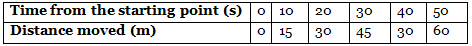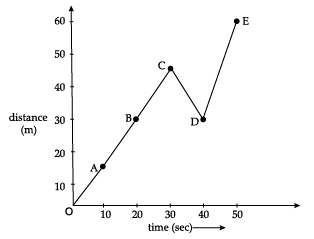Q. What will be the speed if the body travels a distance of 15 metres in 10 seconds?

Detailed Solution for Test: Motion- Case Based Type Questions- 2 - Question 1
1.5 m/s will be the speed if the body travels a distance of 15 metres in 10 seconds.
Test: Motion- Case Based Type Questions- 2 - Question 2

### Direction: The distance moved by a student at different intervals of time, while walking to school, is given in the table below. A distance time graph for the motion of the student is also shown. Study the table and the graph and choose correct options to answer any four questions given below:Q. Name the physical quantities denoted by the slope of the distance–time graph.

Detailed Solution for Test: Motion- Case Based Type Questions- 2 - Question 2

Speed is the physical quantity denoted by slope of distance-time graph.

Test: Motion- Case Based Type Questions- 2 - Question 3

### Direction: The distance moved by a student at different intervals of time, while walking to school, is given in the table below. A distance time graph for the motion of the student is also shown. Study the table and the graph and choose correct options to answer any four questions given below:Q. What does the shape of the graph suggest about the type of motion ?

Detailed Solution for Test: Motion- Case Based Type Questions- 2 - Question 3

As from point A to point C, the student moves with constant speed and from point C to D he is reducing the speed and from D to E again constant speed acceleration.

Test: Motion- Case Based Type Questions- 2 - Question 4

Direction: The distance moved by a student at different intervals of time, while walking to school, is given in the table below.

A distance time graph for the motion of the student is also shown. Study the table and the graph and choose correct options to answer any four questions given below:Q. State a condition under which a body moves in such a way that the magnitude of its average velocity is equal to its average speed.

Detailed Solution for Test: Motion- Case Based Type Questions- 2 - Question 4
The magnitude of average velocity of an object is equal to its average speed if the object moves in a straight line in a particular direction.
Test: Motion- Case Based Type Questions- 2 - Question 5

Direction: The distance moved by a student at different intervals of time, while walking to school, is given in the table below.

A distance time graph for the motion of the student is also shown. Study the table and the graph and choose correct options to answer any four questions given below:Q. An object is said to be moving with ___________ if it covers equal distances in equal intervals of time.

Detailed Solution for Test: Motion- Case Based Type Questions- 2 - Question 5
An object is said to be moving with uniform speed if it covers equal distances in equal intervals of time.
Test: Motion- Case Based Type Questions- 2 - Question 6

Direction: The graph shows the distance-time graph of three objects A, B and C. Study the graph and answer any four questions.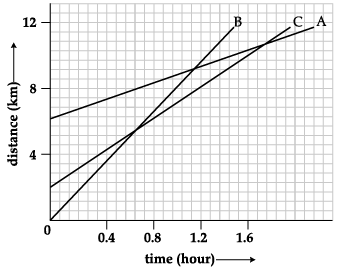Q. How far has B travelled by the time it passes C

Detailed Solution for Test: Motion- Case Based Type Questions- 2 - Question 6
The distance travelled by B is around 5 km.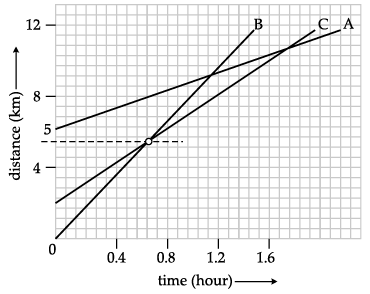Test: Motion- Case Based Type Questions- 2 - Question 7

Direction: The graph shows the distance-time graph of three objects A, B and C. Study the graph and answer any four questions.Q. All the three objects A,B & C __________

Detailed Solution for Test: Motion- Case Based Type Questions- 2 - Question 7
All the three objects A, B and C never meet at a single point. Thus, all the three were never at the same point on the road.
Test: Motion- Case Based Type Questions- 2 - Question 8

Direction: The graph shows the distance-time graph of three objects A, B and C. Study the graph and answer any four questions.Q. Which of the three is travelling the fastest?

Detailed Solution for Test: Motion- Case Based Type Questions- 2 - Question 8

Speed = Distance/Time

From the graph, we see that,

A travels from 6 to 12 km in 2 hours.

B travels from 0 to 12 km in 1.4 hours.

C travels from 2 to 12 km in 1.6 hours.

Since, B travels the most distance, in the smallest time, it is travelling the fastest.

Test: Motion- Case Based Type Questions- 2 - Question 9

Direction: The graph shows the distance-time graph of three objects A, B and C. Study the graph and answer any four questions.Q. How far has C travelled when B passes A?

Detailed Solution for Test: Motion- Case Based Type Questions- 2 - Question 9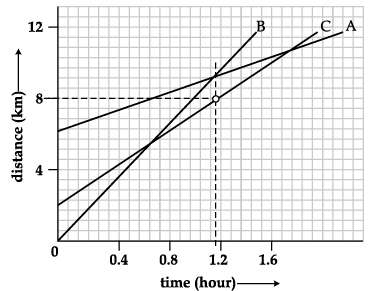The distance travelled by A is 8 km.

Test: Motion- Case Based Type Questions- 2 - Question 10

Direction: The graph shows the distance-time graph of three objects A, B and C. Study the graph and answer any four questions.Q. The distance-time graph is a ______________ when the object is at rest.

Detailed Solution for Test: Motion- Case Based Type Questions- 2 - Question 10
The distance-time graph is a straight line parallel to time axis when the object is at rest.

## Physics for Class 9

16 videos|69 docs|56 tests
Information about Test: Motion- Case Based Type Questions- 2 Page
In this test you can find the Exam questions for Test: Motion- Case Based Type Questions- 2 solved & explained in the simplest way possible. Besides giving Questions and answers for Test: Motion- Case Based Type Questions- 2, EduRev gives you an ample number of Online tests for practice

## Physics for Class 9

16 videos|69 docs|56 tests